Hostname: page-component-594f858ff7-7tp2g Total loading time: 0 Render date: 2023-06-10T16:00:20.227Z Has data issue: false Feature Flags: { "corePageComponentGetUserInfoFromSharedSession": true, "coreDisableEcommerce": false, "corePageComponentUseShareaholicInsteadOfAddThis": true, "coreDisableSocialShare": false, "useRatesEcommerce": true } hasContentIssue false

## Abstract

Previous numerical studies on homogeneous Rayleigh–Bénard convection, which is Rayleigh–Bénard convection (RBC) without walls, and therefore without boundary layers, have revealed a scaling regime that is consistent with theoretical predictions of bulk-dominated thermal convection. In this so-called asymptotic regime, previous studies have predicted that the Nusselt number (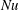$\mathit{Nu}$) and the Reynolds number (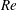$\mathit{Re}$) vary with the Rayleigh number (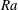$\mathit{Ra}$) according to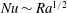$\mathit{Nu}\sim \mathit{Ra}^{1/2}$ and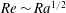$\mathit{Re}\sim \mathit{Ra}^{1/2}$ at small Prandtl numbers (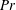$\mathit{Pr}$). In this study, we consider a flow that is similar to RBC but with the direction of temperature gradient perpendicular to gravity instead of parallel to it; we refer to this configuration as vertical natural convection (VC). Since the direction of the temperature gradient is different in VC, there is no exact relation for the average kinetic dissipation rate, which makes it necessary to explore alternative definitions for$\mathit{Nu}$,$\mathit{Re}$ and$\mathit{Ra}$ and to find physical arguments for closure, rather than making use of the exact relation between$\mathit{Nu}$ and the dissipation rates as in RBC. Once we remove the walls from VC to obtain the homogeneous set-up, we find that the aforementioned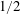$1/2$-power-law scaling is present, similar to the case of homogeneous RBC. When focusing on the bulk, we find that the Nusselt and Reynolds numbers in the bulk of VC too exhibit the$1/2$-power-law scaling. These results suggest that the$1/2$-power-law scaling may even be found at lower Rayleigh numbers if the appropriate quantities in the turbulent bulk flow are employed for the definitions of$\mathit{Ra}$,$\mathit{Re}$ and$\mathit{Nu}$. From a stability perspective, at low- to moderate-$\mathit{Ra}$, we find that the time evolution of the Nusselt number for homogenous vertical natural convection is unsteady, which is consistent with the nature of the elevator modes reported in previous studies on homogeneous RBC.

## JFM classification

Type
JFM Papers
Information
Journal of Fluid Mechanics , 25 April 2018 , pp. 825 - 850

## Access options

Get access to the full version of this content by using one of the access options below. (Log in options will check for institutional or personal access. Content may require purchase if you do not have access.)

## References

Ahlers, G., Grossmann, S. & Lohse, D. 2009 Heat transfer and large scale dynamics in turbulent Rayleigh–Bénard convection. Rev. Mod. Phys. 81, 503537.CrossRefGoogle Scholar
Calzavarini, E., Doering, C. R., Gibbon, J. D., Lohse, D., Tanabe, A. & Toschi, F. 2006a Exponentially growing solutions in homogeneous Rayleigh–Bénard convection. Phys. Rev. E 73, 035301.Google Scholar
Calzavarini, E., Lohse, D., Toschi, F. & Tripiccione, R. 2005 Rayleigh and Prandtl number scaling in the bulk of Rayleigh–Bénard turbulence. Phys. Fluids 17, 055107.CrossRefGoogle Scholar
Calzavarini, E., Toschi, F., Lohse, D., Tripiccione, R., Doering, C. R., Gibbon, J. & Tanabe, A. 2006b Homogeneous Rayleigh–Bénard convection scaling, heat transport and structures. In Proceedings of the Conference and Euromech Colloquium #480 on High Rayleigh Number Convection, Trieste, Italy. ICTP.Google Scholar
Celani, A., Mazzino, A. & Vozella, L. 2006 Rayleigh–Taylor turbulence in two dimensions. Phys. Rev. Lett. 96, 134504.CrossRefGoogle ScholarPubMed
Chillà, F. & Schumacher, J. 2012 New perspectives in turbulent Rayleigh–Bénard convection. Eur. Phys. J. E 35, 125.Google ScholarPubMed
Cholemari, M. R. & Arakeri, J. H. 2009 Axially homogeneous, zero mean flow buoyancy-driven turbulence in a vertical pipe. J. Fluid Mech. 621, 69102.CrossRefGoogle Scholar
Chung, D. & Matheou, G. 2012 Direct numerical simulation of stationary homogeneous stratified sheared turbulence. J. Fluid Mech. 696, 434467.CrossRefGoogle Scholar
Emran, M. S. & Schumacher, J. 2008 Fine-scale statistics of temperature and its derivatives in convective turbulence. J. Fluid Mech. 611, 1334.CrossRefGoogle Scholar
Frick, P., Khalilov, R., Kolesnichenko, I., Mamykin, A., Pakholkov, V., Pavlinov, A. & Rogozhkin, S. 2015 Turbulent convective heat transfer in a long cylinder with liquid sodium. Europhys. Lett. 109, 14002.CrossRefGoogle Scholar
Gibert, M., Pabiou, H., Chillá, F. & Castaing, B. 2006 High-Rayleigh-number convection in a vertical channel. Phys. Rev. Lett. 96, 084501.CrossRefGoogle Scholar
Gibert, M., Pabiou, H., Tisserand, J.-C., Gertjerenken, B., Castaing, B. & Chillá, F. 2009 Heat convection in a vertical channel: Plumes versus turbulent diffusion. Phys. Fluids 21, 035109.CrossRefGoogle Scholar
Grossmann, S. & Lohse, D. 2000 Scaling in thermal convection: a unifying theory. J. Fluid Mech. 407, 2756.CrossRefGoogle Scholar
Grossmann, S. & Lohse, D. 2001 Thermal convection at large Prandtl numbers. Phys. Rev. Lett. 86, 33163319.CrossRefGoogle ScholarPubMed
Grossmann, S. & Lohse, D. 2002 Prandtl and Rayleigh number dependence of the Reynolds number in turbulent thermal convection. Phys. Rev. E 66, 016305.Google ScholarPubMed
Grossmann, S. & Lohse, D. 2004 Fluctuations in turbulent Rayleigh–Bénard convection: the role of plumes. Phys. Fluids 16, 44624472.CrossRefGoogle Scholar
Grossmann, S. & Lohse, D. 2011 Multiple scaling in the ultimate regime of thermal convection. Phys. Fluids 23, 045108.CrossRefGoogle Scholar
Hughes, G. O. & Griffiths, R. W. 2008 Horizontal convection. Annu. Rev. Fluid Mech. 40, 185208.CrossRefGoogle Scholar
Kraichnan, R. H. 1962 Turbulent thermal convection at arbitrary Prandtl number. Phys. Fluids 5, 13741389.CrossRefGoogle Scholar
Lohse, D. & Toschi, F. 2003 Ultimate state of thermal convection. Phys. Rev. Lett. 90, 034502.CrossRefGoogle ScholarPubMed
Lohse, D. & Xia, K.-Q. 2010 Small-scale properties of turbulent Rayleigh–Bénard convection. Annu. Rev. Fluid Mech. 42, 335364.CrossRefGoogle Scholar
Monin, A. S. & Yaglom, A. M. 2007 Statistical Fluid Mechanics: Mechanics of Turbulence, vol. 1. Courier Dover Publications.Google Scholar
Ng, C. S., Ooi, A., Lohse, D. & Chung, D. 2015 Vertical natural convection: application of the unifying theory of thermal convection. J. Fluid Mech. 764, 349361.CrossRefGoogle Scholar
Ng, C. S., Ooi, A., Lohse, D. & Chung, D. 2017 Changes in the boundary-layer structure at the edge of the ultimate regime in vertical natural convection. J. Fluid Mech. 825, 550572.CrossRefGoogle Scholar
Patterson, J. C. & Armfield, S. W. 1990 Transient features of natural convection in a cavity. J. Fluid Mech. 219, 469497.CrossRefGoogle Scholar
Pope, S. B. 2000 Turbulent Flows. Cambridge University Press.CrossRefGoogle Scholar
van Reeuwijk, M., Jonker, H. J. J. & Hanjalić, K. 2008 Wind and boundary layers in Rayleigh–Bénard convection. I. Analysis and modeling. Phys. Rev. E 77, 036311.Google ScholarPubMed
Riedinger, X., Tisserand, J.-C., Seychelles, F., Castaing, B. & Chillá, F. 2013 Heat transport regimes in an inclined channel. Phys. Fluids 25, 015117.CrossRefGoogle Scholar
Schmidt, L. E., Calzavarini, E., Lohse, D., Toschi, F. & Verzicco, R. 2012 Axially homogeneous Rayleigh–Bénard convection in a cylindrical cell. J. Fluid Mech. 691, 5268.CrossRefGoogle Scholar
Sekimoto, A., Dong, S. & Jiménez, J. 2016 Direct numerical simulation of statistically stationary and homogeneous shear turbulence and its relation to other shear flows. Phys. Fluids 28, 035101.CrossRefGoogle Scholar
Shishkina, O. 2016 Momentum and heat transport scalings in laminar vertical convection. Phys. Rev. E 93, 051102.Google ScholarPubMed
Shishkina, O., Grossmann, S. & Lohse, D. 2016 Heat and momentum transport scalings in horizontal convection. Geophys. Res. Lett. 43, 12191225.CrossRefGoogle Scholar
Shishkina, O. & Horn, S. 2016 Thermal convection in inclined cylindrical containers. J. Fluid Mech. 790, R3.CrossRefGoogle Scholar
Shishkina, O., Horn, S., Emran, M. S. & Ching, E.2017 Mean temperature profiles in turbulent thermal convection. Preprint, arXiv:1708.08366.Google Scholar
Smyth, W. D. & Moum, J. N. 2000 Length scales of turbulence in stably stratified mixing layers. Phys. Fluids 12, 13271342.CrossRefGoogle Scholar
Sun, C., Cheung, Y.-H. & Xia, K.-Q. 2008 Experimental studies of the viscous boundary layer properties in turbulent Rayleigh–Bénard convection. J. Fluid Mech. 605, 79113.CrossRefGoogle Scholar
Tisserand, J.-C., Creyssels, M., Gibert, M., Castaing, B. & Chillá, F. 2010 Convection in a vertical channel. New J. Phys. 12, 075024.CrossRefGoogle Scholar
Yu, H., Li, N. & Ecke, R. E. 2007 Scaling in laminar natural convection in laterally heated cavities: is turbulence essential in the classical scaling of heat transfer? Phys. Rev. E 76, 026303.Google ScholarPubMed
Zhou, Q., Stevens, R. J. A. M., Sugiyama, K., Grossmann, S., Lohse, D. & Xia, K.-Q. 2010 Prandtl–Blasius temperature and velocity boundary-layer profiles in turbulent Rayleigh–Bénard convection. J. Fluid Mech. 664, 297312.CrossRefGoogle Scholar